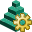# FD Card

This card sets the solver settings for the finite difference time domain (FDTD) solver.

On the Solve/Run tab, in the Solution settings group, click theFDTD settings (FD) icon.

## Parameters:

Automatically determine the time interval to be considered
The time interval is determined automatically by the FDTD solver based on the time signals used by the configuration sources, the size of the computational domain and the material properties. An estimation is made for how much time is required for a pulse to propagate through the whole domain.
Specify the time interval in number of periods
The maximum and/or minimum time interval specified in sinusoidal periods. A period is defined as $\frac{1}{{f}_{centre}}$ , where ${f}_{centre}$ is the average between the upper and lower frequencies in the requested band.
Specify time interval in seconds
The absolute maximum and/or minimum time interval specified in seconds.
Convergence threshold
Convergence threshold for the FDTD solver. The simulation will be terminated if the threshold has been reached and the simulation time is larger or equal to the minimum simulation time (specified or automatically determined).